# Comparing OLPS algorithms on a diversified set of ETFs¶

Let's compare the state of the art in OnLine Portfolio Selection (OLPS) algorithms and determine if they can enhance a rebalanced passive strategy in practice. Online Portfolio Selection: A Survey by Bin Li and Steven C. H. Hoi provides the most comprehensive review of multi-period portfolio allocation optimization algorithms. The authors developed the OLPS Toolbox, but here we use Mojmir Vinkler's implementation and extend his comparison to a more recent timeline with a set of ETFs to avoid survivorship bias (as suggested by Ernie Chan) and idiosyncratic risk.

Vinkler does all the hard work in his thesis, and concludes that Universal Portfolios work practically the same as Constant Rebalanced Portfolios, and work better for an uncorrelated set of small and volatile stocks. Here I'm looking to find if any strategy is applicable to a set of ETFs.

The agorithms compared are:

Type Name Algo Reference
Benchmark CRP Constant Rebalanced Portfolio T. Cover. Universal Portfolios, 1991.
Benchmark UCRP Uniform CRP (UCRP), a special case of CRP with all weights being equal T. Cover. Universal Portfolios, 1991.
Benchmark BCRP Best Constant Rebalanced Portfolio T. Cover. Universal Portfolios, 1991.
Follow-the-Winner UP Universal Portfolio T. Cover. Universal Portfolios, 1991.
Follow-the-Winner EG Exponential Gradient Helmbold, David P., et al. On‐Line Portfolio Selection Using Multiplicative Updates Mathematical Finance 8.4 (1998): 325-347.
Follow-the-Winner ONS Online Newton Step A. Agarwal, E. Hazan, S. Kale, R. E. Schapire. Algorithms for Portfolio Management based on the Newton Method, 2006.
Follow-the-Loser Anticor Anticorrelation A. Borodin, R. El-Yaniv, and V. Gogan. Can we learn to beat the best stock, 2005
Follow-the-Loser PAMR Passive Aggressive Mean Reversion B. Li, P. Zhao, S. C.H. Hoi, and V. Gopalkrishnan. Pamr: Passive aggressive mean reversion strategy for portfolio selection, 2012.
Follow-the-Loser CWMR Confidence Weighted Mean Reversion B. Li, S. C. H. Hoi, P. L. Zhao, and V. Gopalkrishnan.Confidence weighted mean reversion strategy for online portfolio selection, 2013.
Follow-the-Loser OLMAR Online Moving Average Reversion Bin Li and Steven C. H. Hoi On-Line Portfolio Selection with Moving Average Reversion
Follow-the-Loser RMR Robust Median Reversion D. Huang, J. Zhou, B. Li, S. C.vH. Hoi, S. Zhou Robust Median Reversion Strategy for On-Line Portfolio Selection, 2013.
Pattern Matching Kelly Kelly fractional betting Kelly Criterion
Pattern Matching BNN nonparametric nearest neighbor log-optimal L. Gyorfi, G. Lugosi, and F. Udina. Nonparametric kernel based sequential investment strategies. Mathematical Finance 16 (2006) 337–357.
Pattern Matching CORN correlation-driven nonparametric learning B. Li, S. C. H. Hoi, and V. Gopalkrishnan. Corn: correlation-driven nonparametric learning approach for portfolio selection, 2011.

We pick 6 ETFs to avoid survivorship bias and capture broad market diversification. We select the longest running ETF per assset class: VTI, EFA, EEM, TLT, TIP, VNQ . We train and select the best parameters on market data from 2005-2012 inclusive (8 years), and test on 2013-2014 inclusive (2 years).

In :
# You will first need to either download or install universal-portfolios from Vinkler
# one way to do it is uncomment the line below and execute
# or
# !pip install --upgrade -e [email protected]:Marigold/[email protected]#egg=universal-portfolios
#
# if the above fail, git clone [email protected]:marigold/universal-portfolios.git and python setup.py install


Initialize and set debugging level to debug to track progress.

In :
%matplotlib inline

import numpy as np
import pandas as pd
from datetime import datetime
import six
import universal as up
from universal import tools
from universal import algos
import logging
# we would like to see algos progress
logging.basicConfig(format='%(asctime)s %(message)s', level=logging.DEBUG)

import matplotlib as mpl
import matplotlib.pyplot as plt
mpl.rcParams['figure.figsize'] = (16, 10) # increase the size of graphs
mpl.rcParams['legend.fontsize'] = 12
mpl.rcParams['lines.linewidth'] = 1
default_color_cycle = mpl.rcParams['axes.color_cycle'] # save this as we will want it back later

In :
# note what versions we are on:
import sys
print('Python: '+sys.version)
print('Pandas: '+pd.__version__)
import pkg_resources
print('universal-portfolios: '+pkg_resources.get_distribution("universal-portfolios").version)

Python: 2.7.9 |Anaconda 2.2.0 (x86_64)| (default, Dec 15 2014, 10:37:34)
[GCC 4.2.1 (Apple Inc. build 5577)]
Pandas: 0.16.0
universal-portfolios: 0.1


We want to train on market data from 2005-2012 inclusive (8 years), and test on 2013-2014 inclusive (2 years). But at this point we accept the default parameters for the respective algorithms and we essentially are looking at two independent time periods. In the future we will want to optimize the paramaters on the train set.

In :
# load data from Yahoo
# Be careful if you cange the order or types of ETFs to also change the CRP weight %'s in the swensen_allocation
etfs = ['VTI', 'EFA', 'EEM', 'TLT', 'TIP', 'VNQ']
# as later updated here : https://www.yalealumnimagazine.com/articles/2398/david-swensen-s-guide-to-sleeping-soundly
swensen_allocation = [0.3, 0.15, 0.1, 0.15, 0.15, 0.15]
benchmark = ['SPY']
train_start = datetime(2005,1,1)
train_end   = datetime(2012,12,31)
test_start  = datetime(2013,1,1)
test_end    = datetime(2014,12,31)

In :
# plot normalized prices of the train set
ax1 = (train / train.iloc[0,:]).plot()
(train_b / train_b.iloc[0,:]).plot(ax=ax1)

Out:
<matplotlib.axes._subplots.AxesSubplot at 0x1067c2d90>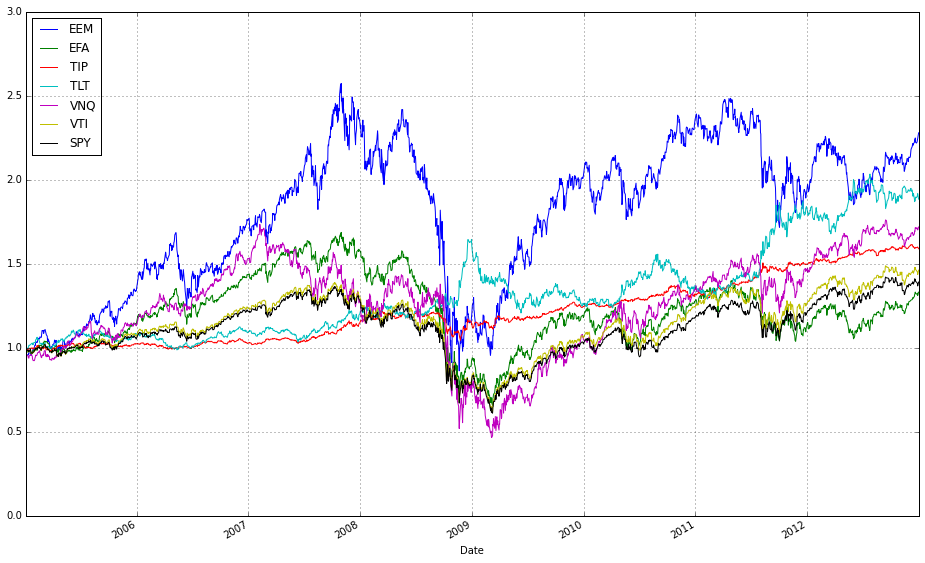In :
# plot normalized prices of the test set
ax2 = (test / test.iloc[0,:]).plot()
(test_b / test_b.iloc[0,:]).plot(ax=ax2)

Out:
<matplotlib.axes._subplots.AxesSubplot at 0x10cbebf10>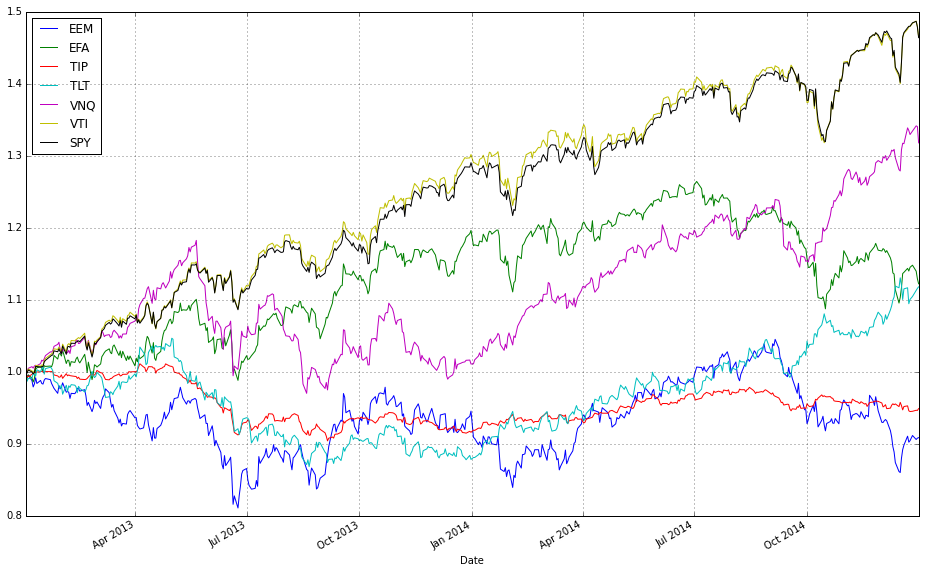# Comparing the Algorithms¶

We want to train on market data from a number of years, and test out of sample for a duration smaller than the train set. To get started we accept the default parameters for the respective algorithms and we essentially are just looking at two independent time periods. In the future we will want to optimize the paramaters on the train set.

In :
#list all the algos
olps_algos = [
algos.Anticor(),
algos.BAH(),
algos.BCRP(),
algos.BNN(),
algos.CORN(),
algos.CRP(b=swensen_allocation), # Non Uniform CRP (the Swensen allocation)
algos.CWMR(),
algos.EG(),
algos.Kelly(),
algos.OLMAR(),
algos.ONS(),
algos.PAMR(),
algos.RMR(),
algos.UP()
]

In :
# put all the algos in a dataframe
algo_names = [a.__class__.__name__ for a in olps_algos]
algo_data = ['algo', 'results', 'profit', 'sharpe', 'information', 'annualized_return', 'drawdown_period','winning_pct']
metrics = algo_data[2:]
olps_train = pd.DataFrame(index=algo_names, columns=algo_data)
olps_train.algo = olps_algos


At this point we could train all the algos to find the best parameters for each.

In :
# run all algos - this takes more than a minute
for name, alg in zip(olps_train.index, olps_train.algo):
olps_train.ix[name,'results'] = alg.run(train)

In :
# Let's make sure the fees are set to 0 at first
for k, r in olps_train.results.iteritems():
r.fee = 0.0

In :
# we need 14 colors for the plot
n_lines = 14
color_idx = np.linspace(0, 1, n_lines)
mpl.rcParams['axes.color_cycle']=[plt.cm.rainbow(i) for i in color_idx]

In :
# plot as if we had no fees
# get the first result so we can grab the figure axes from the plot
ax = olps_train.results.plot(assets=False, weights=False, ucrp=True, portfolio_label=olps_train.index)
for k, r in olps_train.results.iteritems():
if k == olps_train.results.keys(): # skip the first item because we have it already
continue
r.plot(assets=False, weights=False, ucrp=False, portfolio_label=k, ax=ax)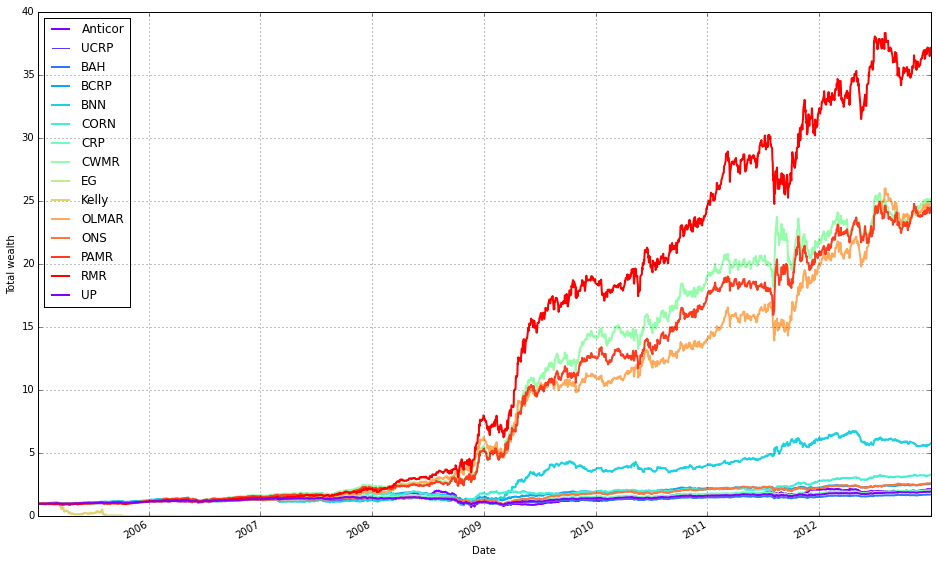In :
def olps_stats(df):
for name, r in df.results.iteritems():
df.ix[name,'profit'] = r.profit_factor
df.ix[name,'sharpe'] = r.sharpe
df.ix[name,'information'] = r.information
df.ix[name,'annualized_return'] = r.annualized_return * 100
df.ix[name,'drawdown_period'] = r.drawdown_period
df.ix[name,'winning_pct'] = r.winning_pct * 100
return df

In :
olps_stats(olps_train)
olps_train[metrics].sort('profit', ascending=False)

Out:
profit sharpe information annualized_return drawdown_period winning_pct
RMR 1.344883 1.417639 1.581531 57.1563 108 55.55556
CWMR 1.30964 1.29867 1.419667 49.69137 134 54.7047
PAMR 1.307667 1.284661 1.405146 49.15968 113 54.55912
OLMAR 1.303725 1.275474 1.358441 49.43 128 54.95495
BNN 1.16316 0.7406326 0.597467 24.49535 516 53.74625
ONS 1.124495 0.5324337 0.4103257 12.69247 301 54.77137
CORN 1.120664 0.5868915 0.3335868 16.03551 619 54.42346
BCRP 1.113103 0.5587799 0.3926754 12.47399 493 52.53479
EG 1.095291 0.4654109 -0.5754063 8.796127 691 54.02584
UP 1.093509 0.4591855 -0.5701021 8.627557 720 54.12525
CRP 1.08585 0.4206153 0.1402307 9.493241 725 54.12525
BAH 1.075043 0.3852003 -0.646195 6.960725 874 54.12525
Anticor 1.06997 0.279532 0.04357109 10.00163 836 53.43968
Kelly 0.8724567 -0.7194364 -0.7692659 -73.22097 2012 51.58002
In :
# Let's add fees of 0.1% per transaction (we pay $1 for every$1000 of stocks bought or sold).
for k, r in olps_train.results.iteritems():
r.fee = 0.001

In :
# plot with fees
# get the first result so we can grab the figure axes from the plot
ax = olps_train.results.plot(assets=False, weights=False, ucrp=True, portfolio_label=olps_train.index)
for k, r in olps_train.results.iteritems():
if k == olps_train.results.keys(): # skip the first item because we have it already
continue
r.plot(assets=False, weights=False, ucrp=False, portfolio_label=k, ax=ax)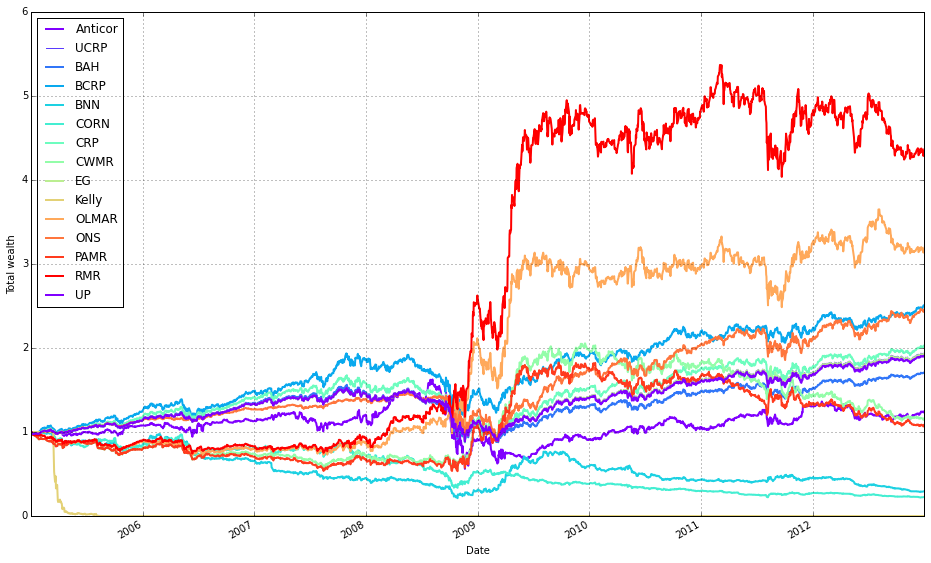### Notice how Kelly crashes right away and how RMR and OLMAR float to the top after some high volatility.¶

In :
olps_stats(olps_train)
olps_train[metrics].sort('profit', ascending=False)

Out:
profit sharpe information annualized_return drawdown_period winning_pct
RMR 1.132369 0.5880135 0.4408147 20.23296 800 51.57107
ONS 1.116896 0.5017011 0.3281846 11.91852 303 54.64481
BCRP 1.110684 0.5474909 0.3639968 12.20745 496 52.50869
OLMAR 1.103785 0.4692946 0.2657746 15.62398 808 50.97354
EG 1.09293 0.4544007 -1.921296 8.579679 720 53.94933
UP 1.091364 0.4491232 -1.002317 8.43104 721 54.04868
CRP 1.083823 0.4110887 0.08867675 9.268793 731 54.09836
BAH 1.074893 0.3844829 -0.6506861 6.947344 874 54.09836
Anticor 1.019879 0.08123565 -0.2464923 2.801569 1108 51.76881
CWMR 1.013093 0.06186626 -0.3053956 1.904562 995 47.28991
PAMR 1.007116 0.03362724 -0.3451079 1.033495 996 46.54401
BNN 0.9001447 -0.5143973 -1.059632 -14.07822 1975 47.01789
CORN 0.8659736 -0.7379091 -1.434188 -16.89046 1975 47.54098
Kelly 0.750211 -1.459831 -1.510671 -93.97284 2012 50.5349

# Run on the Test Set¶

In :
# create the test set dataframe
olps_test  = pd.DataFrame(index=algo_names, columns=algo_data)
olps_test.algo  = olps_algos

In :
# run all algos
for name, alg in zip(olps_test.index, olps_test.algo):
olps_test.ix[name,'results'] = alg.run(test)

In :
# Let's make sure the fees are 0 at first
for k, r in olps_test.results.iteritems():
r.fee = 0.0

In :
# plot as if we had no fees
# get the first result so we can grab the figure axes from the plot
ax = olps_test.results.plot(assets=False, weights=False, ucrp=True, portfolio_label=olps_test.index)
for k, r in olps_test.results.iteritems():
if k == olps_test.results.keys(): # skip the first item because we have it already
continue
r.plot(assets=False, weights=False, ucrp=False, portfolio_label=k, ax=ax)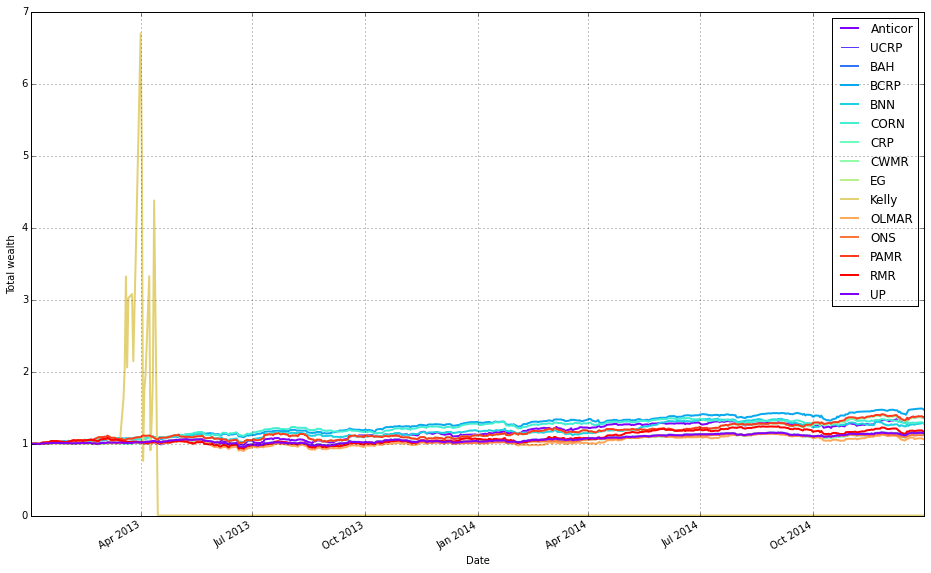### Kelly went wild and crashed, so let's remove it from the mix¶

In :
# plot as if we had no fees
# get the first result so we can grab the figure axes from the plot
ax = olps_test.results.plot(assets=False, weights=False, ucrp=True, portfolio_label=olps_test.index)
for k, r in olps_test.results.iteritems():
if k == olps_test.results.keys() or k == 'Kelly': # skip the first item because we have it already
continue
r.plot(assets=False, weights=False, ucrp=False, portfolio_label=k, ax=ax)